# Using Penalty Points with Different Scoring Types

## Overview

Penalty scoring deducts a value from the student's score for each incorrect response on a Question, and is set by the author in the Question Editor.

Penalty scoring is available on all auto-scored Question types, in the More options section of the Question Editor.

Penalty scoring can behave differently, depending on the Scoring type used in the Question.

## Penalty Scoring with Exact Match

Remember: Exact match requires the student to select all correct response options in a Question to receive the score, otherwise they will receive a score of 0.

In the example below:

• The score for the Question is 3
• The penalty score is 3
• Choice A, Choice B, and Choice C are the correct answers
• Selecting Choice D, either on its own or alongside other options, will give the student a score of 0/3.

Selecting Choice A, Choice B, and Choice C will award the student with a mark of 3/3. Selecting Choice D alongside these options will deduct 3 points from the overall score, leading to a final score of 0/3.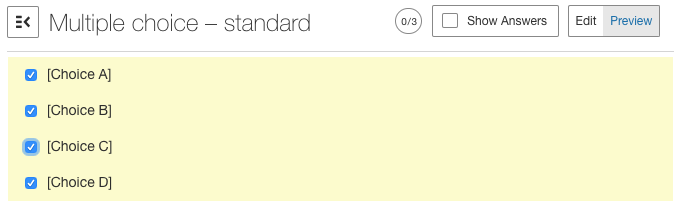Figure 1: Penalty points leading to an overall score of 0.

Selecting Choice A and Choice B will award the student with a score of 0/3, because we are using Exact Match scoring. This means that choosing Choice D, an incorrect option, will not affect the score of 0.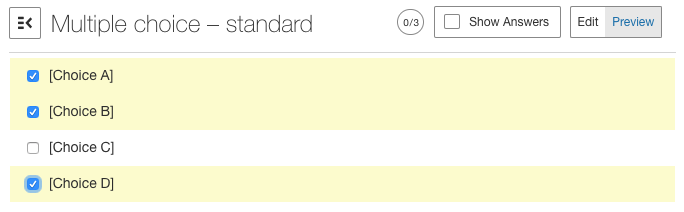Figure 2: Another example of an overall score of 0, unaffected by penalty points.

Selecting only correct response options will award the student with a score of 3/3.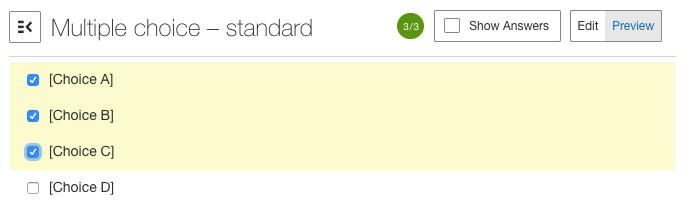Figure 3: Selecting only the correct options will give the student a score of 3.

## Penalty Scoring with Partial Match per Response

Remember: Partial Match per Response will award the student marks for each correct answer they submit on the same Question. The value of each correct answer will match the value entered in the Points field in the scoring section of the Question Editor.

In the example below:

• The score for the Question is 3
• The penalty score is 3
• Choice A, Choice B, and Choice C are the correct answers
• Selecting Choice D, either on its own or alongside other options, will deduct 3 marks from the overall Question score

Selecting only the correct options will give the student a score of 9/9 (each option is worth 3 marks).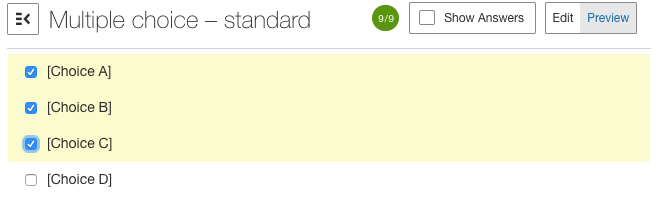Figure 4: Selecting only correct answers means that penalty points will not affect the score.

Selecting only some of the correct options will award the student 3 marks per response, in this case Choice A and Choice B are correct so the student receives 6/9.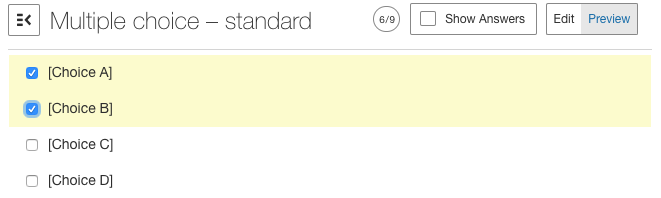Figure 5: Selecting only some correct answers, but no incorrect answers, still means penalty points will not affect the score.

Selecting the three correct options will award the student 9/9 (as each response option is worth 3 marks), but selecting a wrong answer alongside the three correct answers in this case will deduct 3 marks from the overall score. The student will receive a score of 6/9 in this example.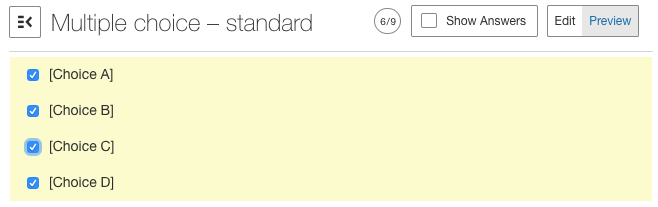Figure 6: Penalty points deducting 3 marks from the overall score.

In this example, selecting two out of three correct answers will award the student with 6 marks out of 9. However, the student has also selected an incorrect answer (Choice D) so they have been deducted 3 marks, leading to an overall score of 3/9.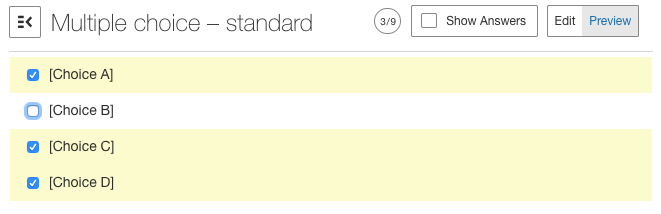Figure 7: Penalty points deducting 3 marks from the overall score again.

## Penalty Scoring with Partial Match

Example 1

Remember: Partial Match will award the student marks for each correct answer they submit on the same Question. The value of each correct answer is calculated by taking the value entered in the Points field in the scoring section of the Question Editor, and dividing that value by the number of correct options on the Question.

In the example below:

• The score for the Question is 3
• The penalty score is 3
• Choice A, Choice B, and Choice C are the correct answers
• Partial Match scoring also applies to the penalty score, I.E. the penalty score is divided by the number of correct options on the Question.
• Therefore, selecting D, on its own or alongside other options, will deduct 1 mark from the overall Question score.
• In this case, the rounding option doesn't make a difference.

Selecting only the correct options will award the student a score of 3/3.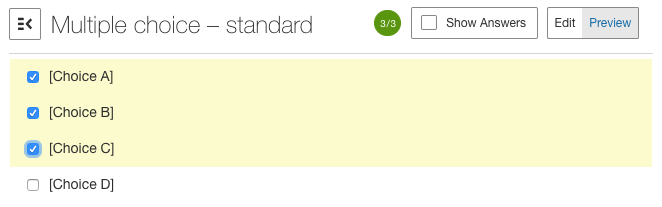Figure 8: Full marks awarded for the Question.

Selecting only some of the correct options will award the student a score of 1 for each correct answer. In this case the student receives a score of 2/3.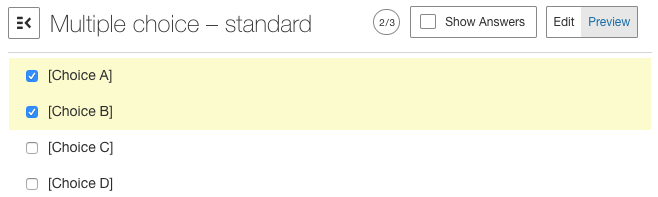Figure 9: Although the student did not receive full marks, penalty points did not affect the score.

Selecting a mixture of correct and incorrect responses will both award and deduct marks. In this example, the student receives 1 mark for each correct answer (Choice A and Choice B), leading to a score of 2/3. The student has also selected an incorrect answer, Choice B, which will deduct 1 mark from the score of 2/3. The student's final score in this example is 1/3.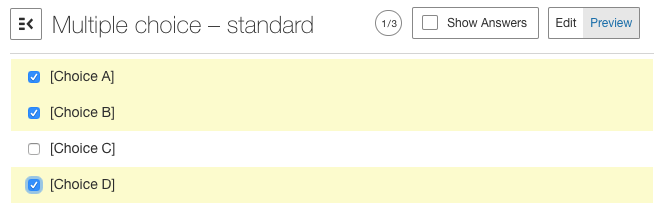Figure 10: Selecting correct and incorrect responses will add to, and deduct from, the overall score.

Selecting all of the correct responses will award the student full marks, 3/3. Selecting an incorrect option alongside these responses will deduct 1 mark from the overall score, leading to a score of 2/3.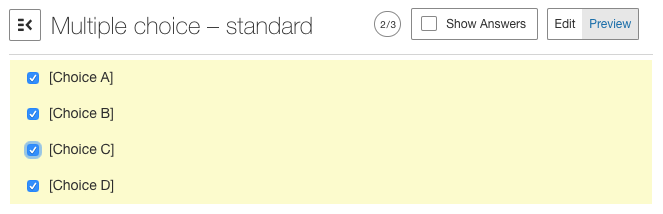Figure 11: Again, selecting correct and incorrect responses will add to, and deduct from, the overall score.

Example 2

In the example below:

• The score for the Question is 3
• The penalty score is 3
• Choice A, Choice B, and Choice C are the correct answers
• Again, remember that Partial Match scoring also applies to the penalty score, I.E. the penalty score is divided by the number of correct options on the Question.
• Therefore, selecting D, on its own or alongside other options, will deduct 2 marks from the overall Question score.
• The value of 2 marks is calculated by applying Partial Match scoring to penalty score of 6, so each correct response is worth 2 marks.
• In this case, the rounding option doesn't make a difference.

Selecting only the correct response options will lead to an overall score of 3/3, because each correct answer is worth 1 mark.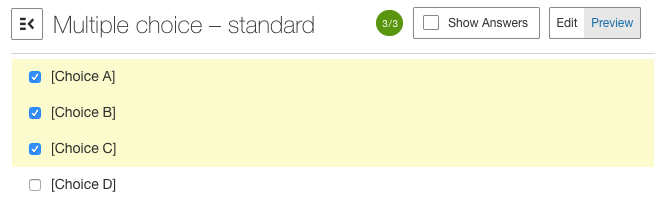Figure 12: Full marks, unaffected by penalty points.

Selecting only some correct answers, but not all, will award the student with 1 mark for each correct answer. In this case, Choice A and Choice B are correct, so the student receives a score of 2/3.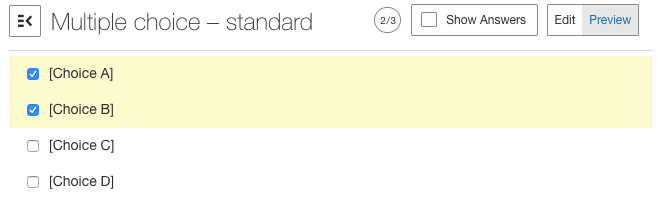Figure 13: Although full marks were not awarded, penalty points did not affect the score.

Selecting a mixture of correct and incorrect options will both award and deduct the student marks. In this example, the student receives 1 mark for each correct answer (Choice A and Choice B), leading to a score of 2/3. Because Choice D has also been selected, 2 points will be deducted from the overall score, leading to a final score of 0/3.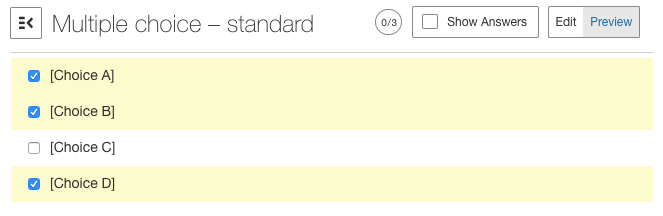Figure 14: Selecting a mixture of correct and incorrect options will add to, and subtract from, the overall score.

In this example, a mixture of incorrect and correct options have been selected again. In this case, Choice A, Choice B, and Choice C all carry 1 mark each, leading to a score of 3/3. Because Choice D, an incorrect option, has also been selected, 2 marks will be deducted from the overall score. The final score is 1/3.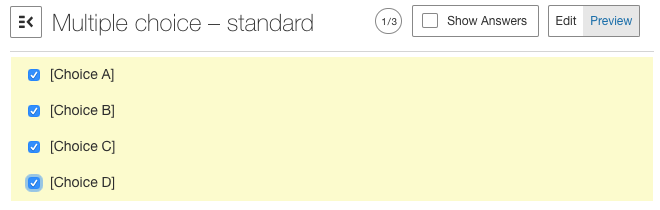Figure 15: Again, selecting a mixture of correct and incorrect options will add to, and subtract from, the overall score.